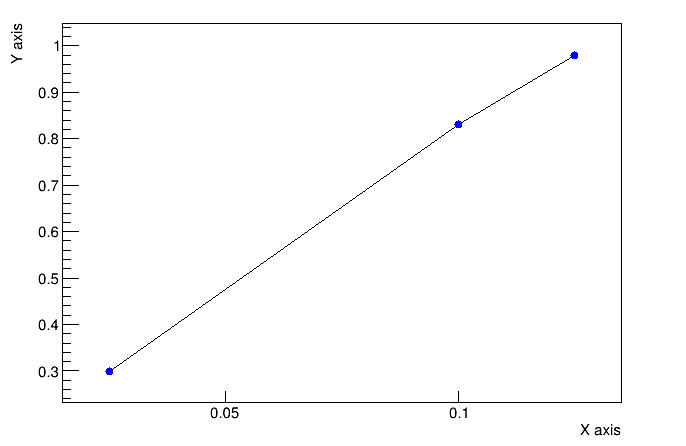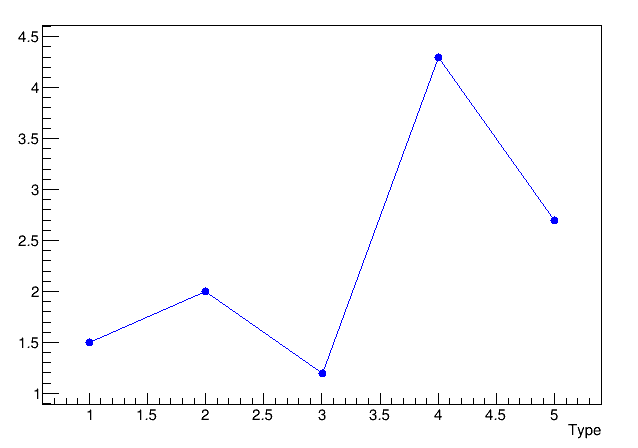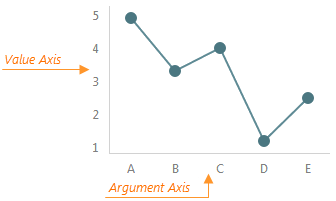# Draw X-Axis with specific values

Hello, I would like to plot three non-uniform points 0.025 0.1 0.125, I am trying to display on the X-axis only the points 0.025 0.1 0.125 or display a string value (0.5x0.5x0.1 0.5x0.5x0. 4 0.5 x0.5x0.5) respectively.
I had tried with the examples from the Forum but I could not find a good solution.

test.C

``````void test()
{

TGraph *g1 = new TGraph("aa.txt");
g1->SetMarkerStyle(20);
g1->SetMarkerColorAlpha(kBlue, 0.35);
g1->GetYaxis()->SetTitle("Y axis");
g1->GetXaxis()->SetTitle("X axis");
g1->SetMarkerSize(1);
TAxis *axis = g1->GetXaxis();
axis->SetNdivisions(5, kTRUE);
g1->Draw("APL");
}
``````

aa.txt

``````0.025 0.3
0.1   0.83
0.125 0.98
``````_
__

ROOT Version: Not Provided
Platform: Not Provided
Compiler: Not Provided

This is a slight modification of a macro found on this forum some time ago, so all credit goes to the original author, couet:

``````void aa()
{
TGraph *g1 = new TGraph("aa.txt");
g1->SetMarkerStyle(20);
g1->SetMarkerColorAlpha(kBlue, 0.35);
g1->GetYaxis()->SetTitle("Y axis");
g1->GetXaxis()->SetTitle("X axis");
g1->SetMarkerSize(1);

g1->Draw("APL");
g1->GetXaxis()->SetLabelSize(0);
g1->GetXaxis()->SetTickLength(0);

Int_t i,n;
Double_t x,y;
TLatex *t;
TLine *tick;
TLine *grid;
double ymin = g1->GetHistogram()->GetMinimum();
double ymax = g1->GetHistogram()->GetMaximum();
double dy = (ymax-ymin);
n = g1->GetN();
for (i=0; i<n; i++) {
g1->GetPoint(i,x,y);
t = new TLatex(x, ymin-0.03*dy, Form("%4.3f",x));
t->SetTextSize(0.03);
t->SetTextFont(42);
t->SetTextAlign(21);
t->Draw();
tick = new TLine(x,ymin,x,ymin+0.03*dy);
tick->Draw();
grid = new TLine(x,ymin,x,y);
grid->SetLineStyle(3);
grid->Draw();
}
}
``````

You can leave out the “grid” lines if not needed.
Edit - Original post:

thank you dastudillo It works well

Hello dastudillo now i have another question
I have 5 points in the X axis, each corresponding to a type eg A, B, C, D, E respectively. how can display A, B, C, D, E in X axis
Best regards
a.txt

``````1 1.5
2 2
3 1.2
4 4.3
5 2.7
````````````#include <stdio.h>

void a(){
FILE *f = fopen("a.txt","r");

char line;
float x,y;
auto g = new TGraph();
int i=0;
while (fgets(line,160,f)) {
sscanf(line  ,"%g %g",&x,&y);
g->SetPoint(i,y,x);
i++;
}
fclose(f);
g->Draw("AL*");
}

``````
1 Like

thank you couet for the reply,
I think I misexplained my question, The question is to display in X axis a string A, B, C, D, E, each for this string corresponding to a line in the a.txt file (see example in image below) .
Best regards``````void a(){
auto g = new TGraph("a.txt");
g->Draw("AL*");

TAxis *X = g->GetXaxis();
X->SetNdivisions(9);
X->ChangeLabel(1,-1,-1,-1,-1,-1,"A");
X->ChangeLabel(2,-1,-1,-1,-1,-1,"B");
X->ChangeLabel(3,-1,-1,-1,-1,-1,"C");
X->ChangeLabel(4,-1,-1,-1,-1,-1,"D");
X->ChangeLabel(5,-1,-1,-1,-1,-1,"E");